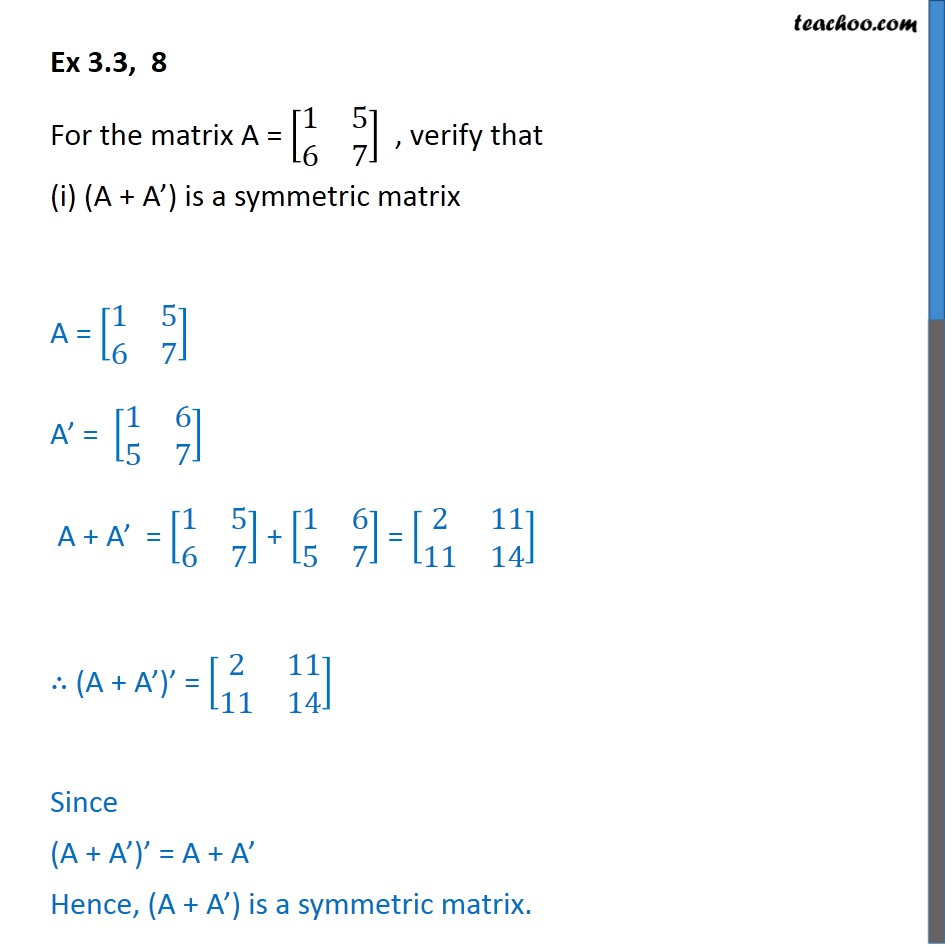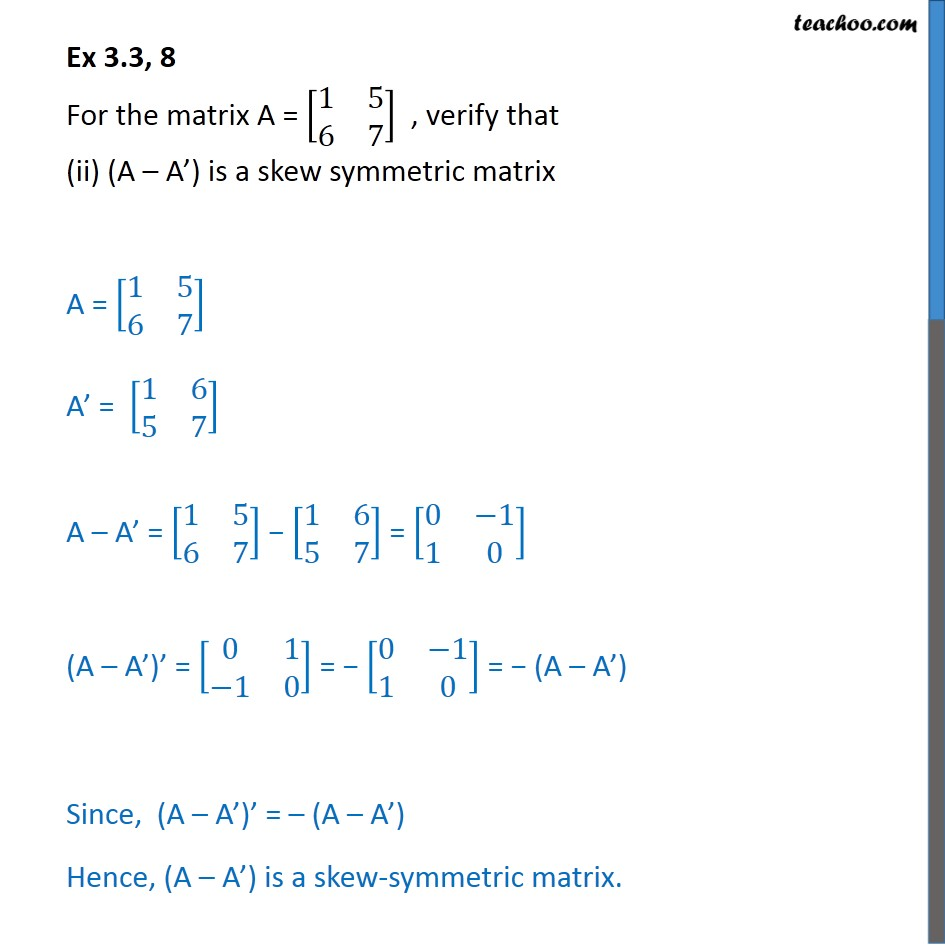Ex 3.3

Chapter 3 Class 12 Matrices (Term 1)
Serial order wise### Transcript

Ex 3.3, 8 For the matrix A = [■8(1&5@6&7)] , verify that (i) (A + A’) is a symmetric matrix A = [■8(1&5@6&7)] A’ = [■8(1&6@5&7)] A + A’ = [■8(1&5@6&7)] + [■8(1&6@5&7)] = [■8(2&11@11&14)] ∴ (A + A’)’ = [■8(2&11@11&14)] Since (A + A’)’ = A + A’ Hence, (A + A’) is a symmetric matrix. Ex 3.3, 8 For the matrix A = [■8(1&5@6&7)] , verify that (ii) (A – A’) is a skew symmetric matrix A = [■8(1&5@6&7)] A’ = [■8(1&6@5&7)] A – A’ = [■8(1&5@6&7)] − [■8(1&6@5&7)] = [■8(0&−1@1&0)] (A – A’)’ = [■8(0&1@−1&0)] = − [■8(0&−1@1&0)] = − (A – A’) Since, (A – A’)’ = – (A – A’) Hence, (A – A’) is a skew-symmetric matrix.